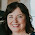## Sunday, May 19, 2013

### Pythagorean Theorem

Tomorrow, I will be teaching a lesson on the Pythagorean Theorem.  I wanted to let the students actually see why it worked.  However, with the school year so close to ending and the end of the year events canceling more math classes than I can spare, I was stumped how to do it.

As you can see, I was able to put together a graphic organizer for them.  The best part of this organizer was taken from Jennie's idea at mathfoldables.blogspot.com.  She had this nifty way of showing students that the sum of the square of the legs is equal to the square of the hypotenuse.  That proof makes up the upper left hand corner.

The formula and the transposed formulas to find the length of the legs are in the upper right hand corner.  The bottom left is example problems and the bottom right is an explanation of Pythagorean Triples and two examples.The template for Pythagorean Theorem proof can be found at http://www.teacherspayteachers.com/Product/Pythagorean-Thereom-Proof-Foldable-Template-496569.  It is a free download.  The students color each sides a different color.Then under the hypotenuse side, the students color 16 squares the same color they did for side b and 9 squares in the same color they did for side a.  What they will see is that their are enough squares to hold all of side a and side b.

1.This graphic organizer is exactly what I need for my Pythagorean Theorem unit I am going to be teaching next month. Thank you for sharing it.

2.Do you have a copy of the graphic organizer?

3.I love this! Can you share the graphic organizer?

4.